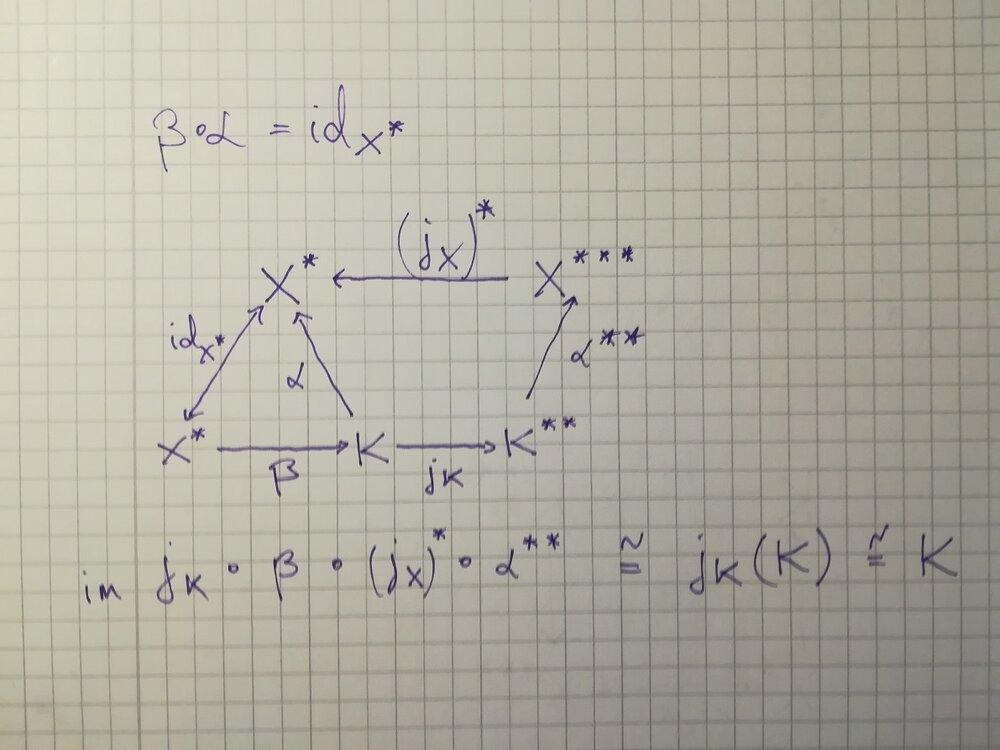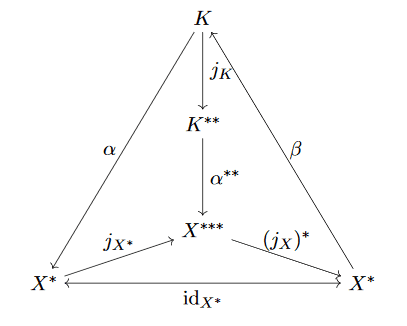Math Challenge - July 2020

• Challenge
• Featured
etotheipi
12. Given a positive integer in decimal representation without zeros. We build a new integer by concatenation of the number of even digits, the number of odd digits, and the number of all digits (the sum of the former two). Then we proceed with that number.
Determine whether this algorithm always comes to a halt. What is or should be the criterion to stop?

I don't really know if this is what you're after, but for any given integer with 4 or more digits there will be either at least 2 even digits or at least 2 odd digits, and the next pass of the algorithm will always reduce the length of that string by at least one digit. You can just keep doing this until you get down to 3 digits, which are either

##\{\text{even}, \text{even}, \text{even}\} \implies 303 \implies 123##
##\{\text{even}, \text{even}, \text{odd}\} \implies 213 \implies 123##
##\{\text{even}, \text{odd}, \text{odd}\} \implies 123##
##\{\text{odd}, \text{odd}, \text{odd}\} \implies 033 \implies 123##

And I guess if you start off with an integer with 1 or 2 digits, the next pass gives you a 3 digit number and we're back to the above.

Last edited by a moderator:
Mentor
2021 Award
I don't really know if this is what you're after, but for any given integer with 4 or more digits there will be either at least 2 even digits or at least 2 odd digits, and the next pass of the algorithm will always reduce the length of that string by at least one digit. You can just keep doing this until you get down to 3 digits, which are either

##\{\text{even}, \text{even}, \text{even}\} \implies 303 \implies 123##
##\{\text{even}, \text{even}, \text{odd}\} \implies 213 \implies 123##
##\{\text{even}, \text{odd}, \text{odd}\} \implies 123##
##\{\text{odd}, \text{odd}, \text{odd}\} \implies 013 \implies 123##

And I guess if you start off with an integer with 1 or 2 digits, the next pass gives you a 3 digit number and we're back to the above.
I had a more formal argument in mind, but ok. I think you mistyped 013. Also I guess is a bit thin. And you could have actually answered the questions. However, these are only formal deficits.

•nuuskur, member 587159 and etotheipi
Point of reference
Assume for a contradiction $C[0,1]$ is isomorphic to a dual Banach space.

Take a sequence of functions with norm $1$ in $C[0,1]$ and with pairwise disjoint support. For instance, pick a sequence of pairwise disjoint intervals in $[0,1]$ and pick a function whose support is that interval. The linear span of this sequence is dense in $c_0$: match the sequence with the canonical basis in $c_0$.

The space $C[0,1]$ is separable. By Theorem 3.1 (Sobczyk), the closed copy of $c_0$ is complemented in $C[0,1]$. By assumption $c_0$ is complemented in a dual Banach space. Dual Banach spaces are complemented in their second dual, therefore $c_0$ is complemented in its second dual, which is $\ell _\infty$. But this contradicts Theorem 3.2 (Phillips-Sobczyk), by which $c_0$ can't be complemented in $\ell _\infty$. Thus, $C[0,1]$ can't be isomorphic to a dual Banach space.

Last edited:
wrobel
I have already stuck at here:
Take a sequence of functions with norm in and with pairwise disjoint support. For instance, pick a sequence of pairwise disjoint intervals in and pick a function whose support is that interval. The linear span of this sequence is dense in : match the sequence with the canonical basis in .

I have already stuck at here:

Firstly, we want the sequence to be linearly independent, hence pairwise disjoint supports. So, denote it $f_n\in C[0,1],\ n\in\mathbb N$. We also want an isometry, so pick the $f_n$ with norm $1$ i.e make sure $f_n$ reaches the unit sphere. Let the $f_n$ span the subspace $K\subseteq C[0,1]$. Then put $\varphi (f_n) = e_n\in c_0$ where $e_n = (\underbrace{0,\ldots,1}_{n},0,\ldots),\ n\in\mathbb N$. The disjointness of supports guarantees we have an isometry.
Let $a:=\sum _{k=1}^\infty \lambda _ke_k\in c_0$ and $\varepsilon >0$. Take $N\in\mathbb N$ such that $\left \|\sum _{k>N} \lambda _ke_k \right \| <\varepsilon$. So $a$ is close to $\varphi \left ( \sum _{k=1}^N \lambda _kf_k \right )$. Now $\overline{\varphi (K)} = c_0$ implies $\overline{K} \cong c_0$.

wrobel
ok
By assumption is complemented in a dual Banach space.

ok
Remark. Formally, the terminology is ".. is a complemented subspace of ..", but I'm used to saying " .. is complemented in ..".

Let a closed subspace $K\subseteq X^*$ be complemented in $X^*$. In other words, there exist continuous maps $\alpha : K \to X^*$ and $\beta : X^* \to K$ such that $\beta\circ \alpha = \mathrm{id}_{K}$. Denote $j_X :X\to X^{**}$ the canonical embedding, then we have a projection $j_{K}\circ\beta\circ (j_X)^*\circ\alpha ^{**}$ onto $j_K(K)\cong K$. One can show the following diagram (there's a typo - it should read $\beta\circ \alpha = \mathrm{id}_K$) is commutative..it's a routine check, but it takes some time and I'm too tired right now.

Remark. Formally, the terminology is ".. is a complemented subspace of ..", but I'm used to saying " .. is complemented in ..".

Let a closed subspace $K\subseteq X^*$ be complemented in $X^*$. In other words, there exist continuous maps $\alpha : K \to X^*$ and $\beta : X^* \to K$ such that $\beta\circ \alpha = \mathrm{id}_{K}$. Denote $j_X :X\to X^{**}$ the canonical embedding, then we have a projection $j_{K}\circ\beta\circ (j_X)^*\circ\alpha ^{**}$ onto $j_K(K)\cong K$.
Alright, I'm recharged now.This diagram is commutative. In particular $(j_X)^* \circ \alpha ^{**} \circ j_K = \alpha$, therefore $j_K \circ \beta \circ (j_X)^* \circ \alpha ^{**}$ is a projection onto $j_K(K) \cong K$ i.e $K$ is complemented in its second dual. It suffices to check $\alpha^{**}\circ j_K = j_{X^*} \circ \alpha$, because the transpose $(j_X)^*$ is left inverse to the embedding $j_{X^*}$ (the bottom subtriangle commutes). Fix $k\in K$ and $x^{**}\in X^{**}$. Then we have the equalities
\begin{align*} (j_{X^*} \circ \alpha)(k)(x^{**}) &=j_{X^*}(\alpha (k))(x^{**}) \\ &= x^{**}(\alpha(k)) \\ &=\alpha ^* (x^{**})(k) \\ &= j_K(k)(\alpha ^*(x^{**})) \\ &=\alpha ^{**}(j_K(k))(x^{**}) \\ &= (\alpha ^{**} \circ j_K)(k)(x^{**}). \end{align*}
In particular, every dual Banach space is complemented in its second dual. This fact is also directly proved in the notes I linked in #128.

Last edited:
wrobel
By assumption is complemented in a dual Banach space.
In which dual space c_0 is complemented? By which assumption?

In which dual space c_0 is complemented? By which assumption?
The assumption is $C[0,1]$ (which is separable) is isomorphic to a dual Banach space $X^*$. By Sobczyk's theorem $c_0$ is complemented in $X^* \cong C[0,1]$.

wrobel
Oh now I see. It looks correct. But by the Krein–Milman theorem it would be easier

I understand the idea, but I haven't worked through a proof of Krein-Milman, so it's a bit uncomfortable language. On the other hand, I am very comfortable with diagram chasing :)

@Math_QED Perhaps, you've been busy or you just missed #125.

Last edited:
member 587159
@Math_QED Perhaps, you've been busy or you just missed #125.

Yes, sorry for the delay in a couple of days I will have time to look at your post.

•nuuskur